# Automata, algebraic theory of

(diff) ← Older revision | Latest revision (diff) | Newer revision → (diff)

A branch of the theory of automata (cf. Automata, theory of) in which algebraic tools are employed in the study of automata. The algebraic theory of automata is based on the fact that automata can be regarded as special algebras or algebraic systems. In addition, events which are representable by finite automata form an algebra with respect to the operations of union, composition and iteration, generated by a finite set of so-called elementary events, each one of which consists of a one-letter or empty word. The algebraic approach makes it possible to utilize algebraic results in the theory of automata directly, and in certain cases aids in establishing a connection between the theory of automata and other branches of mathematics. E.g., the theory of automata was used to obtain a proof of the solvability of certain second-order arithmetical theories, and also to obtain a new, simpler solution of the Burnside problem.

From the algebraic point of view, a finite or infinite automata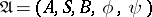is a tribasic algebra, i.e. an algebra with three sets of elementsandand two operations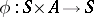and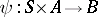. On the other hand, a transition system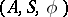, whereis the input alphabet,is the set of states (cf. Automaton, finite) can be regarded as an algebra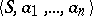with unary operations, denoted by the letters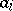of the input alphabet, such that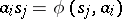. Concepts such as a homomorphism of automata (cf. Automata, homomorphism of), an isomorphism, a sub-automaton, etc., as applied to automata, are thus naturally defined. This approach also permits the assignment to the automatonof a semi-group of transformations of the set(under the composition operation), generated by the operations, so that for any arbitrary,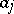fromandfromone puts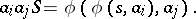This semi-group is known as the semi-group of the automatonand is used as a means of describing certain properties of automata (classification, decomposability, isomorphism, etc.) in algebraic language. Also, to any semi-group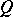with a unit element there may be assigned an automaton with a given input alphabet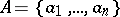and a set of statesas follows. To each letterofis assigned some elementfrom, and the transition function is then defined as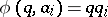. The semi-group of such an automaton is isomorphic to the sub-semi-group ofgenerated by the elements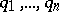, which means that ifare generators of, then the semi-group of the automaton is isomorphic to the original semi-group. The semi-group of an automaton is clearly isomorphic to a quotient semi-group of the input semi-group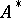of all words over the alphabetwith the operation of successive union (concatenation) of words according to the congruenceFor any statethe congruence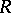is the maximal subcongruence of the relationThis means, in particular, that an event which can be represented by the initial acceptoris a union of certain-classes. Since the semi-group of an automaton characterizes it up to an isomorphism, various classes of semi-groups have their own classes of automata. If the semi-group of an automaton is free, Abelian, cyclic, nilpotent, etc., or is itself a group, the automaton is known, respectively, as a free, Abelian, cyclic, nilpotent or a group automaton; these last are also called permutation automata. Another approach, connected with the algebraic classification of transition and output functions, leads to the classes of linear, substitution and other automata (cf. Automaton). Substitution automata realize one-to-one functions and are used in coding theory. Linear automata are of interest because of their simple structure.

An automatonis said to be a linear automaton if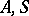andare linear spaces over some field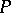,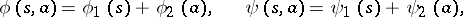where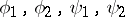are, respectively, linear mappings ofinto,into,into, andinto. It is usually assumed that the fieldis finite, and that the spacesandare finite-dimensional; in such a case a linear automaton is a finite automaton. If multiple operations are permitted in the representation of a finite acceptor as an algebra whose operations are letters of the input alphabet, the resulting generalization is known as an automaton over terms (automaton over trees; generalized automaton). Such automata are used to prove the solvability of certain second-order mathematical theories.